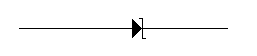# Test: Electronic Devices & Circuits - 6

## 25 Questions MCQ Test Analog Electronics | Test: Electronic Devices & Circuits - 6

Description
Attempt Test: Electronic Devices & Circuits - 6 | 25 questions in 25 minutes | Mock test for Electrical Engineering (EE) preparation | Free important questions MCQ to study Analog Electronics for Electrical Engineering (EE) Exam | Download free PDF with solutions
QUESTION: 1

Solution:
QUESTION: 2

Solution:
QUESTION: 3

### Consider the following statements Acceptor level lies close the valence band.Donor level lies close to the valence band.n type semiconductor behaves as an insulator at 0 K.p type semiconductor behaves as an insulator at 0 K. Of these statements:

Solution:
QUESTION: 4

If the temperature of on extrinsic semiconductor is increased so that the intrinsic carrier concentration is doubled, then

Solution:
QUESTION: 5

Assertion (A): The conductivity of an n type semiconductor increases with increase in temperature and increase in density of donor atoms.

Reason (R): Diffusion of carriers occurs in semiconductors.

Solution:
QUESTION: 6

No load d.c. output will be least in case of

Solution:
QUESTION: 7

When an electron breaks a covalent bond and moves away,

Solution:
QUESTION: 8

In which condition does BJT behave like a closed switch?

Solution:
QUESTION: 9

A photo diode is

Solution:
QUESTION: 10

Germanium and Si phosphorus have their maximum spectral response in the

Solution:
QUESTION: 11

No load d.c. output will be least in case of

Solution:
QUESTION: 12

When an electron breaks a covalent bond and moves away,

Solution:
QUESTION: 13

A photo diode is

Solution:
QUESTION: 14

In which condition does BJT behave like a closed switch?

Solution:
QUESTION: 15

Germanium and Si phosphorus have their maximum spectral response in the

Solution:
QUESTION: 16

High purity copper is obtained by

Solution:
QUESTION: 17

Photo electric emission can occur only if the frequency of light is more than threshold frequency.

Solution:
QUESTION: 18

In a JFET the width of channel is controlled by

Solution:
QUESTION: 19

The unit of thermal resistance of a semi-conductor device is

Solution:
QUESTION: 20

Figure represents aSolution:
QUESTION: 21

For generating 1 MHz frequency signal, the most suitable circuit is

Solution:
QUESTION: 22

A doped semi-conductor is called

Solution:
QUESTION: 23

In n type MOSFET, the substrate

Solution:
QUESTION: 24

The first and the last critical frequency of an RC driving point impedance function must respectively by

Solution:
QUESTION: 25

The diffusion current is proportional to

Solution:Use Code STAYHOME200 and get INR 200 additional OFF Use Coupon Code